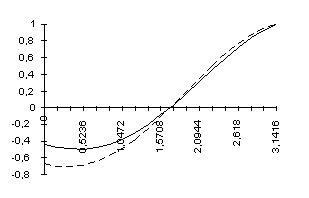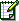A Simplified Study of GIT

Created on 08-16-98 - JLN Labs - Last update 08-19-98

Let’s denote A (rear part) the point where the track is widest (and where the engine is), and B (front part) the opposite point where the track is narrowest. Due to this variable width, the ball is continuously exchanging angular momentum against linear momentum. At point B its linear speed is maximum et its spinning RPM is low, while at opposite point A, it is the other way round : translation speed is low with an important spin, still enhanced by the motor. It appears immediately that such a movement of the ball is producing on the frame two effects opposite to one another. Namely :

an effect due to centrifugal force. As the average speed of the ball during the half turn B is much higher than during half turn A it clearly generates a forward force

an effect due to accelerations et decelerations of the ball (tangentiel effect). When the shrinking track forces the ball to gain linear speed thus loosing angular momentum, the ball is taking support from the track, which generates a rearward force equal to the product of ball mass by the acceleration it undergoes. Clearly, during the path from A to B, such a force, always tangent to the trajectory, is rearward. During the return path from B to A, there is a braking of the ball supported by the track, thus generating anew a force tangent to the trajectory rearward as well. The resulting effect is then the difference between these two effects. Using a few simplifying assumptions, the calculation is easy. We then asume :

• that the system is kept motionless

• that the trajectory of the ball is a circle (nearly the case)

• that the track is made in such a way that the ball speed varies linearly from A to B and conversely from B to A (this is always possible) and that there is no slipping of the ball on track.

• that there is no friction, which allows to neglect the engine. If the frame is kept motionless, clearly the return path BA will be exactly symmetrical of path AB, thus generating the same forces and allowing a calculation on the first half only.

Notations

R radius of (circular) path of the center of the ball

r instantaneous (variable) roliling radius of the ball

a angle defining ball position, starting from origin A anticlockwise

v linear speed (variable) of the ball.

V maximum linear speed of the balle at point B

r ratio of minimum linear speed of the ball at point A to the maximum speed at point B

Dynamics of a ball rolling on a variable width track

Kinetic energy of the ball has two components : translation energy and spin the sum remaining constant if friction is neglected. If m is the mass of the ball, I its inertia and w its spinning speed, we then have

mv2 + Iw2 = Constant

but if r is the (variable) rolling radius at a given time, we have w = v/r, so

(m + I/r2)v2 = Constant

On the other hand, as the ball is hollow, its thickness can be neglected, so its inertia I is equal to 8p/3.h4, h being the radius of the ball. Expressed as a function of the ball mass m = 4ph2 such inertia writes 2/3.mh2 , so that, suppressing m, we can write :

(1+ 2/3.h2/r2)v2 = Constant

In our case, with a track width of 35 mm in A, the rolling radius rA of the ball is 9,7 mm and reaches in B a value rB = 19,6 mm for a track width of 8 mm. It is then easy to get the ratio v2/V2 which is :

(1+ 2/3.h2/rB2)/(1+ 2/3.h2/rA2)

the end result being v2/V2 = 0,442, that is v/V = r = 0,665

Computation of centrifugal force

The linear speed v varies from Vr (mini) to V (maxi) according to the formula

v = Vr + a/p.(V - Vr) = V [r + a/p.(1 - r)]

which genetates a centrifugal force equal to (ball mass is taken as equal to unity)

v2/R = V2/R.[r + a/p.(1 - r)]2

which must be multiplied by cos a to get the projection on axis AB

Computation of track reaction

Ball movement being uniformly accelerated from V1 in A to V2 en B, the tangential (constant) acceleration during this course of a length of pR equals (V2-V2r2)/2pR and its component on axis AB is

V2(1-r2)/2pR.sin a

Summing up the two forces

it may be noted that the radius of the ball does not appear in any of these two calculations. The resulting thrust on a half turn as a function of a, then writes :

V2/R.{[r + a/p.(1 - r)]2 .cos a + (1-r2)/2p.sin a}

Giving to r the value calculated above, this expression becomes :

V2/R.{[0,665 + a/p.0,335]2 .cos a + 0,558/2p.sin a}

or, giving its value to p

V2/R.{[0,665 + 0,106.a]2 .cos a + 0,089.sin a}

The curve below (continuous line. Vertical scale has been inverted to get positive values for forward thrusts) represents the thrust as a function of a.. The area of the right part being greater than the area of the left part, this could be taken as a proof of a positive resulting thrust, but, as the orbital velocity is not constant the integration should not be made with respect of the angle a but rather, as pointed out by Cyril Smith, with respect of time. Before integration, he last expression must then be divided by da/dt = (0,665 + 0,106.a). It becomes

- (0,665 + 0,106.a) .cos a + 0,089.sin a / (0,665 + 0,106.a)giving the curve in dotted line whose total resultant area is equal to zero, the right part being equal to the left part, at least to the accuracy of the Excel spreadsheet. The rearward thrust being applied during a longer period of time than the forward thrust, it can be understood that the end result amounts to zero.

Conclusion

This confirms the conclusions of Hans Holzherr and Charles North that can be found at

http://www.geocities.com/ResearchTriangle/1986/GIT/GITconclusion.html

Quoting them, I would say contrary to my first conclusion :

If the GIT works it does so because of some non-Newtonian principle which requires demonstrating and formulating.Email to Jean de Lagarde: jlagarde@cyberaccess.fr

Sujet : Excelent math for dumb weight slingers, now let's try to analyze the TORQUE components
Date : 18/08/1998 21:18:26
From:
davidc@open.org (David E. Cowlishaw)
To: jlagarde@cyberaccess.fr

Jean de Lagarde;

Thanks for your interest and efforts on behalf of the GIT! I reviewed
both your and Charles North (with Hans Holzherr)'s math derivations, and
quite agree with you that the sum of the "tug of war" created by the
stronger centrifugal balanced by the two opposing tangential accelerations
will be zero.

That's as it should be, and several now have lent their own proofs to
that integration over time.

However, as of today, few have considered the torque components
generated by the orbitals on the total sum of forces, perhaps thinking that
the interaction and shift of linearly expressed momentum (as centrifugal
force) into the NON LINEARLY considered spin accelerations of the orbitals,
has no bearing on reality. Assumptions that are fatal to an accurate
examination!

The torque generated on the orbital as the inverse acceleration
exchange is taking place, has a counter torque on the system of course, and
here is where the "magic" translation of angular acceleration to linear
takes place!

The tangential accelerations of the orbital NOW pair off a portion of
their momentum exchange with the race as your analysis details, and a
portion of that equally expressive radial momentum "disappears" from the
center of system mass (which includes the linearly considered mass of that
orbital, since spinning or non-spinning, it's linear mass doesn't change),
around the axis of the orbital. Convieniently, it apparently happens to be
pairing off with the tangential forces (also angular in two opposing
halves) giving us momentum that is stored "in another dimension".

Try to think of it this way, although the outside of the orbital (and
it's center of mass) is decelerating and pushing back on the race, the
wheel is accelerating the inside of the orbital (it's center of turning
sees more wheel surface pass when it slows it's forward velocity), "pushing
off" in the forward direction from ANGULAR mass, and on the return cycle,
the unwinding spin also pushes forward on the center wheel, while the net
zero sum of tangential and centrifugal is tilted in centrifugal's faver IN
THIS DIMENSION (linear acceleration), because we can only have so much
energy "combatting" or pairing off with the centrifugal. The torque
coupling of the two bilatterally opposed torque expressions (angular
orbital spin torque, pairing with orbital or tangential torque) of
neccessity "steals" the linearly expressable momentum, "swallowing" it as
spin momentum and releasing it in a (mostly ;) single direction as the
cycle completes.

Find the vectors of the orbital spin torque acceleration's input,
realize that the momentum in the spin is subrtacted from SOMEWHERE as a
moving mass interaction, and a more accurate math portrayal of MY device,
rather than a dumb mass whirler will be achieved.

ANYway, thanks again for your efforts, the zero sum of centrifugal and
tangential is quite needed to give us a baseline proof, then it can be
shown that the actual centrifugal force imbalance derives from the
"external mass" interaction with the (seperate or outside) angular mass
property of the orbitals, since any momentum the orbital spin axis contains
is removed (and returned in a reinforcing direction) from your linearly
considered system mass, and the time and position in the cycle that the
momentum is NOT linearly interacting but winding around a traveling,
spinning orbital, is time the positive portions of the cycle can gain

Follow the momentum rather than the mass, you'll find that it does
indeed result in an exchange of angular acceleration for linear
acceleration! The total sums may be zero and your proof valid, lacking
only the break out of the angularly force-paired portion of the tangential
interaction stored as spin accelerations, which would show the now
unpaired (linearly) direction of the centrifugally expressed thrust so many
are finding in reality, contrary to current science dogma.

Remember also that centrifugal force is the square of velocity, and
though extra time is spent on the race at lower velocities at the tail,
time is merely ADDITIVE, and the sum total of centrifugal only, all the way
around the race, is still tugging forward, the zero sum difference is the
orbital's acceleration and deceleration against the center of system mass,
known as the tangential force opposed pair (our magic "hermaphrodite" force
expression mediator, being both linear AND angular!), that completes the
"dumb" or non-spinning orbit mass variable orbit velocity machine, and
results in a net zero for that machine (not the GIT).

Just a few thoughts here as I psych myself up to do the next update
containing the Mars Con info, gotta go, talk with you later!

18 August 1998 - CC: Matheads Group; Jean-Louis Naudin
Reference:
http://ourworld.compuserve.com/homepages/jlnaudin/html/GITanal.htm

David Eugene Cowlishaw